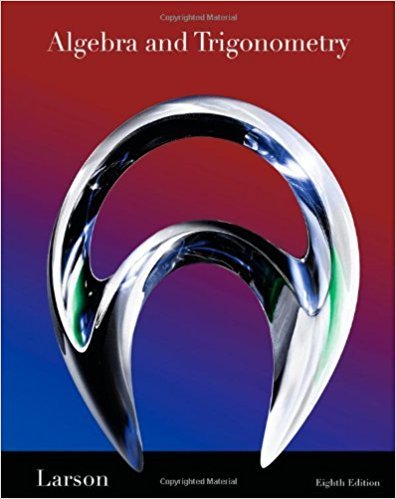×
×

# Solutions for Chapter 6.3: Trigonometric Functions of Any Angle## Full solutions for Algebra and Trigonometry | 8th Edition

ISBN: 9781439048474Solutions for Chapter 6.3: Trigonometric Functions of Any Angle

Solutions for Chapter 6.3
4 5 0 426 Reviews
20
1
##### ISBN: 9781439048474

Since 120 problems in chapter 6.3: Trigonometric Functions of Any Angle have been answered, more than 46971 students have viewed full step-by-step solutions from this chapter. This textbook survival guide was created for the textbook: Algebra and Trigonometry, edition: 8. Algebra and Trigonometry was written by and is associated to the ISBN: 9781439048474. Chapter 6.3: Trigonometric Functions of Any Angle includes 120 full step-by-step solutions. This expansive textbook survival guide covers the following chapters and their solutions.

Key Math Terms and definitions covered in this textbook
• Circulant matrix C.

Constant diagonals wrap around as in cyclic shift S. Every circulant is Col + CIS + ... + Cn_lSn - l . Cx = convolution c * x. Eigenvectors in F.

• Column space C (A) =

space of all combinations of the columns of A.

• Companion matrix.

Put CI, ... ,Cn in row n and put n - 1 ones just above the main diagonal. Then det(A - AI) = ±(CI + c2A + C3A 2 + .•. + cnA n-l - An).

A sequence of steps (end of Chapter 9) to solve positive definite Ax = b by minimizing !x T Ax - x Tb over growing Krylov subspaces.

• Distributive Law

A(B + C) = AB + AC. Add then multiply, or mUltiply then add.

• Free variable Xi.

Column i has no pivot in elimination. We can give the n - r free variables any values, then Ax = b determines the r pivot variables (if solvable!).

• Hankel matrix H.

Constant along each antidiagonal; hij depends on i + j.

• Linearly dependent VI, ... , Vn.

A combination other than all Ci = 0 gives L Ci Vi = O.

• Minimal polynomial of A.

The lowest degree polynomial with meA) = zero matrix. This is peA) = det(A - AI) if no eigenvalues are repeated; always meA) divides peA).

• Nullspace matrix N.

The columns of N are the n - r special solutions to As = O.

• Orthonormal vectors q 1 , ... , q n·

Dot products are q T q j = 0 if i =1= j and q T q i = 1. The matrix Q with these orthonormal columns has Q T Q = I. If m = n then Q T = Q -1 and q 1 ' ... , q n is an orthonormal basis for Rn : every v = L (v T q j )q j •

• Rank one matrix A = uvT f=. O.

Column and row spaces = lines cu and cv.

• Reduced row echelon form R = rref(A).

Pivots = 1; zeros above and below pivots; the r nonzero rows of R give a basis for the row space of A.

• Right inverse A+.

If A has full row rank m, then A+ = AT(AAT)-l has AA+ = 1m.

• Rotation matrix

R = [~ CS ] rotates the plane by () and R- 1 = RT rotates back by -(). Eigenvalues are eiO and e-iO , eigenvectors are (1, ±i). c, s = cos (), sin ().

• Solvable system Ax = b.

The right side b is in the column space of A.

• Standard basis for Rn.

Columns of n by n identity matrix (written i ,j ,k in R3).

• Trace of A

= sum of diagonal entries = sum of eigenvalues of A. Tr AB = Tr BA.

• Vector v in Rn.

Sequence of n real numbers v = (VI, ... , Vn) = point in Rn.

• Volume of box.

The rows (or the columns) of A generate a box with volume I det(A) I.

×